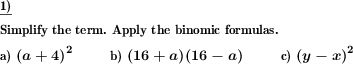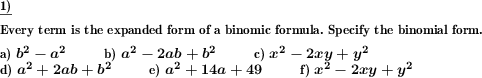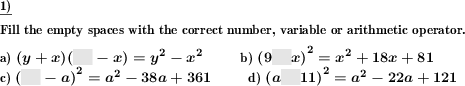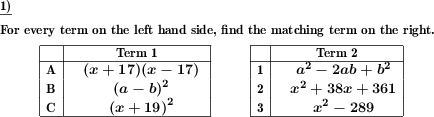Custom math worksheets at your fingertips# Details for problem "Expand and simplify binomic formula"

Quickname: 7813

Junior High School, Middle School, High School.

## Summary

Apply binomic formula expansion rules to simplify term.

## Example## Description

Apply the well known binomic formula expansion rules to expand and simplify terms.

The summands of the formula's factors will be either a variable and a literal number or both of them will be variables. In no case, both summands will be numbers, which means the expanded term will always contain variables and it does not simplify to a single literal number.

The number of problems is selectable. For the types of formulas that will appear it can be specified that only some of the three binomic formulas will be used.

Variables that appear will always be named a and b or x and y.

Download free printable worksheets for this math problem here. The worksheet contains the problems only, the solution sheet includes the answers. Just click on the respective link.

•Worksheet 1Solution sheet with answers
•Worksheet 2Solution sheet with answers
•Worksheet 3Solution sheet with answers

If you can not see the solution sheets for download, they may be filtered out by an ad blocker that you may have installed. If this is the case, please allow ads for this page and reload the page. The solution sheets will then reappear.

• Do these sample worksheets do not really fit?
• Do you need more math worksheets, with a different level of difficulty?
• Would you like to combine different problems on a worksheet and adjust them to your needs?
• As a teacher, you can put together your own worksheets using the automatically generated math problems provided.
With a free initial credit, you can start creating your own math worksheets in a few minutes.

You can try it for free! Register here, to create custom worksheets now!

## Customization options for this problem

Parameter
Possible values
Number of problems
1, 2, 3, 4, 5, 6, 7, 8, 9, 10
Binomic formula types
(a+b)^2, (a-b)^2, (a+b)(a-b), (a+b)^2 & (a-b)^2, all

## Similar problems

Remark
Description
Fill in blank spaces in binomic formulas
Fill gaps correctly in binomic formulas
Get to know the elements of binomic formulas
Elements of a binomic formula and their expanded form are grouped in a table. Fill the empty spaces.
Simpler version
Apply binomic formula expansion rules to simplify term. Advanced version mit multiples of variables as summands.
Matching problem: Find correct pairs of binomic formulas and expanded terms
Find matching terms of original and simplified binomic formulas in two lists.
Expand and simplify a simple term
Expand product term with variables.
Binomic formula is embedded in other term
Simplify a term which contains a binomic formula
The other way round: Specify the binomic formula
A term is given which is the expanded form of a binomic formula. The original binomic formula has to be found.

## dw-Math worksheet templates that do contain this problem

Name
Title
Description
Binomic formulas: Expand and simplify
This worksheet provides basic exercises to become familiar with the binomic formulas.

## Other types of problems that appear on worksheets with this problem:

Relevance
Name
Description
Quickname
Example
****
Specify binomic formula for expanded term
A term is given which is the expanded form of a binomic formula. The original binomic formula has to be found.****
Binomic formula fill in blanks
Fill gaps correctly in binomic formulas****
Match binomic formula to simplified form
Find matching terms of original and simplified binomic formulas in two lists.